Relaxation Oscillations in Floating Liquid Bridges | OMICS International
Chemical Sciences Journal

# Relaxation Oscillations in Floating Liquid Bridges

Teschke O*, Gomes WE, Roberto de Castro J, Filho JFV and Soares DM

Laboratório de Nanoestruturas e Interfaces, Instituto de Física, UNICAMP, 13083-859, Campinas, SP, Brazil

*Corresponding Author:
Teschke O
Laboratório de Nanoestruturas e Interfaces
Instituto de Física, UNICAMP, 13083-859
Campinas, SP, Brazil
Tel: 55 (19) 3521-4148
Fax: 55 (19) 3521-5637
E-mail: [email protected]

Received date July 08, 2015; Accepted date July 09, 2015; Published date July 15, 2015

Citation: Teschke O, Gomes WE, Roberto de Castro J, Filho JFV, Soares DM (2015) Relaxation Oscillations in Floating Liquid Bridges. Chem Sci J 6:099. doi:10.4172/2150-3494.100099

Copyright: © 2015 Teschke O, et al. This is an open-access article distributed under the terms of the Creative Commons Attribution License, which permits unrestricted use, distribution, and reproduction in any medium, provided the original author and source are credited.

Visit for more related articles at Chemical Sciences Journal

#### Abstract

A floating liquid bridge configuration that operates in a pulsed relaxation mode in which charge (cations) and fluid injection alternatively assume forward and opposite directions in the bridge structure is reported. Cations formed at the high voltage anode are surrounded by clusters of dimethylsulphoxide (DMSO) molecules that migrate to the cathode resulting in forward fluid transport with a period of ~200 s until an equilibrium point is attained. The fluid motion then assumes the opposite direction of the current for~60 s. Charged clusters travel to the bridge surface modifying its structural properties forming a plastic layer with an ~85 KPa stiffness.

#### Keywords

Relaxation oscillator; Charged DMSO clusters; Fluid and charge injection; Charged fluid interface; High-voltage dielectric fluid conduction

#### Introduction

The interaction between a dielectric liquid and an electric field has produced a phenomenon that defies intuition as the formation of a free-standing fluid bridge when electric fields of 106 V/m are applied between two capillaries separated by a distance of ~10 mm . When the bridge is broken, small liquid jets from an anodic beaker are ejected and accompanied by spark discharges [2,3]. This phenomenon is an extension of the Taylor cone , in which a fluid forms a stable liquid structure floating in air [5,6].

After the water bridge was rediscovered in 2007 [2,7], this structure was assumed to exhibit properties that were similar to the properties of a polymer melt  or hydrogen bonds were assumed to be the driving force of bridge formation . In the computer simulation, a structure was formed if the electric field was at least ~103 times stronger than the electric field required for the formation of macroscopic structures in real experiments [2,10-16]. Some attempts have been made to reveal its specific microstructure by neutron scattering and Raman scattering [10,11,15] however, various explanations of the stability has been offered [3,17-20], questions remains about the mechanisms responsible for the bridge structure; an overview of liquid bridges is given by Wexler et al. . Because the phenomenon of liquid bridging is not restricted to water it can be observed in other liquids. The problem is subsequently connected with the dynamics of a dielectric fluid , which is important for capillary jets  in printers and electrosprays [23,24]. Consequently, the nonlinear dynamics of the flow of free surfaces has been intensively examined [25,26]. To investigate the mechanisms involved in the floating liquid bridge formation, which remain unknown, another polar dielectric fluid dimethylsulphoxide (DMSO) was investigated because of its high electrical permittivity associated with its low conductivity.

After bridge formation, the transport of water between the two reservoirs was observed; an equilibrium situation was investigated in our previous study , in which the levels remain stationary by adding and removing liquid from the reservoirs. In the present study in order to correlate current and fluid flow a configuration that generates observable oscillations of both charge and liquid was build. Pulsed charge injection was investigated in connection with the liquid displacement in the bridge structure, and the relaxation oscillation periods were measured.

#### Experimental Part

The floating DMSO bridge apparatus consisted of two beakers with an attached capillary, as shown in Figure 1a; it is filled to the top edge of the capillaries with DMSO, as shown in Figure 1b and the lower edge shown in Figure 1c. DMSO is a polar liquid insulator with a conductivity of 0.002 μS/cm and a relative permittivity of ~47. We employed DMSO from Merck®; its impurities primarily consisted of water with a concentration < 0.04%. A bridge is formed between two glass capillaries. For the capillaries in contact, liquid drops continuously fall at the connection region. When a voltage is applied between the two beakers, dripping stops. The first beaker was fixed and the second beaker was mounted to a 3D translational stage. Beakers were placed to ensure that the capillary tubes were initially aligned and in contact with one another. The bridge was formed by applying a high voltage between two platinum wire electrodes, with their lateral surfaces isolated and immersed in the liquid in each beaker. A high-voltage DC power supply was employed (Vmax ~25 kV); its negative terminal was grounded. The current source was formed by this power supply in series with the high internal fluid resistance in the beakers. The current was typically limited to ~50 μA. The capillaries made contact and one beaker was slowly translated from the other end of the capillary beaker. The temperature of the liquid in the beakers was measured. The floating liquid bridge relaxation oscillations were repeatedly observed. The dynamics of the current and flow transition have been investigated by optical microscopy and by measuring the current and fluid volume time dependency. The essential dynamic features of this experiment were registered as images obtained with a digital CCD color TopWay camera at a rate of 30 frames per second for various separations and applied voltages.

Figure 1: (a) Optical image of the water bridge setup showing the two beakers with capillary tubes, (b) high and (c) low liquid level at the capillary connection are indicated by arrows and (d) floating bridge diameter for three stages: top image depicts the forward flux, middle image depicts the flux from the cathode to the anode (referred to as reverse) and in lower image the end of the forward direction period occurs where the lack of liquid in the capillaries decreases the diameter of the bridge.

#### Results and Discussion

The pulsed response of the floating bridge configuration to an applied voltage of 13 kV is shown in the optical image in Figure 1d. The flux occurs in the forward direction in the top image. The middle image depicts the fluid bridge in the stage in which the fluid flux direction extends from cathode to anode (referred to as reverse), whereas the lack of liquid in the capillaries decreases the diameter of the bridge, which indicates the end of the forward flux period (bottom image).

The electrical response of the floating bridge configuration to a high applied voltage is a current pulse train, as shown in Figure 2 (the current is given by the full line). For a 13 kV applied voltage, the current oscillates from a minimum value of ~12 μA to a maximum value of ~35 μA. The liquid flow was measured by registering the fluid height variation in both beakers (refer to the scale in Figure 1a).

The oscillations, as indicated in (Figure 2), were observed in a configuration that begins with equilibrated liquid levels in both beakers when a 13 kV potential difference is applied; after a few cycles (~3), a quasi-steady state is attained. A positive current pulse is indicated by stage 1 in Figure 2; associated with this pulse, fluid is transported from anode (+) to cathode (-), which is observed by an increase in the liquid level in the cathode reservoir. The current decreases over time until it attains a minimum value, which is depicted by the low liquid level at the anode capillary indicated in Figure 1c. The extra length in the current circuit introduces a high resistance in series with the bridge structure, which results in a decrease in carrier injection and a lower voltage across the bridge structure; fewer charges are present in the bridge and its surface. At this instant, the pressure generated by the higher level of the liquid at the cathode induced a backflow, which is indicated by stage 2 and shown in Figure 2 by the dashed line curve. A positive current of ~12 μA is observed at the backflow stage, and the fluid flows in the inverse direction (see stage 3 in Figure 2). The pressure difference induced by gravity due to the height difference is sufficient to drive the fluid to the anode in this high resistance bridge stage. As the anode reservoir fills to the original level, the thin fluid layer at the beaker wall and inside the capillary disappear and the drift induced by the high electric field reverses the cycle, i.e., charge and fluid both flow from anode to cathode (stage 4 in Figure 2) and the cycle restarts. The flow from the anode to the cathode lasts ~200 s but flux reversion only lasts for ~60 s. Because the transferred volume in both half cycles is equal, the reverse flux velocity is higher than the velocity of the liquid induced by the electric field. Fluid loss occurs by dripping in the reverse flux interval; thus, the current pulse amplitude is not repetitive.

Figure 2: Current vs time curve (full line) for two capillary tubes separated by 5 mm and for a 13 kV applied voltage to the bridge electrodes. The dotted line shows the variation in liquid height in the cathode reservoir as a function time.

DMSO is an aprotic solvent and the highest concentration of impurities corresponds to water, so in order to investigate the contribution of H+ and OH- ions in the conduction process, water was added to DMSO. The effect of 10% w/w addition is shown in Figure 3. No substantial changes in the current oscillating profile characterized by a pulse periodicity (period increased by a factor of 2) and minimum and maximum current (decreased by a factor of 1.6) values were introduced by water addition. The initial water concentration in DMSO was <0.04% (maximum impurities in DMSO) by adding water, the concentration of water was increased by a factor of ~250.

Figure 3: Current vs time for a liquid bridge formed with a 10% Milli-Q added to DMSO; the spatial configuration is identical to the configuration shown in Figure 2.

The conductivity of the liquid is given by the number of charge carriers. In water we have thermal generated carriers H+ and OH-; in the bridge another type of carrier is generated at the high voltage electrode, for voltages ≥4 kV the high voltage generated cations play the dominant role. Consistent with this statement is the charging of the cathode fluid reservoir top surface observed when we operate the bridge in continuous mode by maintaining the equilibrium of the fluid levels in both beakers . If the current conduction were associated with the H+ and/or OH- ions transport, an appreciable increase in current should be observed. What we have measured in the DMSO matrix is that the addition of the impurity water decreases the conduction, decreasing the charge transport. The addition of water at high voltages results in a decrease in the conductivity probably because it interfere the p+ mobility.

We propose that the basic mechanisms involved in the dynamics of the relaxation oscillations in liquid floating bridges are as follows: (a) cations are generated at the Pt electrode/liquid interface by the high electric field for applied voltages greater than ~4 kV. This cations radially orient neighbor molecules by forming clusters; water clusters have been previously reported [27,28]. The size of the cluster may be estimated by equating the thermal energy of the liquid kT to the cluster electric energy eφ, where φ is the electric potential of a point charge, e is the electron charge, k is the Boltzmann constant and T is the absolute temperature. The radius of the solvated arrangement calculated by this relation is r=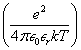and is equal 55 nm for εr ~1 (~7 nm for εr ~8). The applied longitudinal electric field induces a slight dipole formation in these cation clusters (Figure 4). The calculated dipole moment of the ion cluster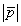=2re is 1.78×10-26 C∙m (0.22×10-26 C∙m for εr=8). For an applied potential of 13 kV, the electric field inside the liquid bridge for a bridge span of 1.0 cm is 13×105 V/m, resulting an orientational energy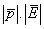of 23×10-21 J for εr=1 (2.86×10-21 for εr=8), larger or of the order of the thermal energy kT=4×10-21 J. The applied electric field is then capable of enforcing the alignment of the dipole moment cation clusters; thus, the induced large dipole clusters will attract one another and they have a tendency to stick together and increasing the stability of the bridge structure, as shown in (Figure 5). In order to form an aligned structure of only DMSO molecules it is necessary an electric field of ~109 V/m. The applied electric field is ~105 V/m so no alignment should be observed. Our point is that we have generated cations which align radially the DMSO molecular dipoles up to a distance of ~55 nm for εr=1 (~7 nm for εr=8) forming clusters which only need 105 V/m to be aligned. If they are observed by neutron scattering or X-ray diffraction no aligned is to be observed since the alignment in the clusters is radial. The relative permittivity of DMSO is εr ≈ 47 but in the previous paragraph we have used εr ≈ 1. This is related to the fact that close to ions, molecules are aligned rigidly resulting in a relatively permittivity of εr ≈ 1 as ~discussed in our previous work for liquid/air interfaces . In order to evaluate this effect, Table 1 shows for various values of the cluster permittivity the calculated cluster radius r from the equilibrium equation KT=eφ. A simple calculation shows that we are not far from equilibrium because the applied electric field (E ~106 V/m) in the cluster with r ~55 nm is substantially smaller than the radial field of the cation. So kT ~eφradial can be used to estimate the cluster radius. A schematic diagram of the cluster distribution in the bridge structure is shown in Figure 4. Water tetrahedral arrangements were also reported to be oriented . The electric field produced by the cation orients the DMSO molecules as far as ~55 nm (for εr ~1) but, DMSO dipoles are much less aligned at the end of the cluster so the external electric field aligns preferentially the microscopic dipole arrangement outer layer. (b) Solvated cations are transported to the cathode, which is observed by an increase in the liquid level at the cathode reservoir. To identify the mechanism involved in the backflow, we have calculated the fluid velocity in the bridge (bridge internal radius of 0.17 mm), where the fluid displacement is induced by the difference in fluid height in the reservoirs. From the Bernoulli equation, we determine that the fluid velocity is given by v=(2gh)1/2, where h is the height difference in the liquid level and g is the gravity acceleration. The calculated velocity for h=2 mm from the Bernoulli equation is ~0.35 m/s. From the value shown in Figure 2 for the last cycle, we obtain a fluid velocity of 0.27 m/s, in reasonable agreement with the calculated velocity. This value of the fluid velocity compared well with the value measured by Woisetschlager et al. . For 60 seconds, the flux is inverted and the cycle restarts.

Figure 4: Schematic diagram of the floating liquid bridge charge and molecular dipoles distribution, longitudinal and transversal cross section.

Figure 5: The calculated bulk modulus of the bridge as a function of the bridge outer layer thickness. The arrow indicates the point of the probable inner layer radius of 50 μm.

εr r (nm)(10-26C∙m)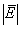(105 V/m)
1 55.60 1.78 2.3
5 11.12 0.36 11.5
8 6.95 0.22 18.0
47 1.18 0.04 1000.0

Table 1: Cluster radius for various dielectric constant around the ionic charge and the corresponding dipole moment of the cluster and the applied electric necessary to orient this clusters, i.e.,≥ KT.

Table 2 shows the values of the time constant involved in the observed relaxation oscillations. The time constant ranges from ~50 s to 200 s. These values are substantially larger than the time constant for the current relaxation in electric circuits. Here, ionized molecules migrate in the insulating dielectric fluid by the action of the electric field, and in the backflow period, neutral molecules return by gravity; thus, the involved relaxation processes are associated to a charged fluid transport.

Fluid Current rise time(seconds) Current fall time(seconds) DMSO flux injection time constant(seconds) DMSO inverse flux time constant(seconds)
DMSO 31.3 170.0 206.3 60.0
DMSO 40.3 193.5 233.9 250.0
DMSO + 10% H2O v/v 35.0 365.5 - - - - - - - - - -

Table 2: Electrical and mechanical time constant involved in the floating liquid bridge relaxation oscillations.

Relaxation oscillations are a type of limit cycle , in the floating liquid bridge the current limit is attained when the pressure associated with the difference in liquid level Δhpg is equal to the electric force/ area, i.e., (neμ|E|An important observation is that a positive current is present when a liquid flux in the opposite direction is observed. There are then two types of liquid carriers: neutral molecules responsible for the reverse flux and charged clusters that are moved by the applied electric field and form the forward flux. Because the positively charged molecules repel one another, they migrate to the liquid/air interface where they modify the interfacial tension and are responsible for the new mechanical properties of the bridge structure. If we assume that the charged layer is plastic and not liquid, the bulk modulus value of the bridge structure is calculated as follows: If an elongation is produced by the weight of the water mass (m) in a cylinder of length (l), radius (r) and produced flexure s, the Young’s modulus (M) [30,31] is given)/A , where n is the ion concentration and μ is the ion mobility.

by the following equation. Because the ionized molecules are mostly restricted to the bridge surface, the expression of bending of a hollow tube with external radius rout and internal radius rin was employed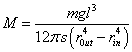This high-voltage formed structure thickness is difficult to measure. Thus, we have plotted the Young´s modulus as a function of rin, as shown in Figure 5, and observe that a substantial increase in bulk modulus value is observed for internal radii larger than 0.5 mm (rout=0.56 mm) . We have concluded that the plastic structure has a thickness smaller than 50 μm. The bulk modulus for this thickness is 85 KPa, which is substantially higher than the value calculated for a full cylinder . This layer moves with a constant velocity along the longitudinal bridge. For a 10 μA current, rout=0.56 mm, and a wall thickness of 50 μA, the corresponding current density is ~5.6 mA/cm2. The calculated values for the wall thickness, the electric field intensity inside the bridge plastic structure and the induced dipole moment describe a possible scenario for the operation of the floating liquid bridge.

#### Conclusion

It is shown that cation generated at the anode alter the liquid molecular distribution forming clusters with a radius ~55 nm for εr=1 (~7 nm for εr=8) given by the thermal equilibrium expression (kT ~φe). The formation of the DMSO floating bridge is the result of the transport of clusters of DMSO surrounding cations formed at the anode electrode. Charged clusters that travel to the liquid interface modify the mechanical properties of the floating bridge structure. The transition flow to backflow has been directly observed by monitoring the current and the fluid volume variation in both the cathode and anode deposits. The measured time constant ranges from ~50 s to 200 s. These values are substantially larger than the time constant for the current relaxation in electric circuits. In the direct flow, the cation drift motion at the outer surface is observed, for a fluid flow in the opposite direction, a net positive electric current in the outer layer remains. These results show that a 2D plastic-like outer layer and a 3D liquid-like inner region form the floating liquid bridge structure. The mechanical stability expression gives the maximum thickness of the outer layer and the minimum corresponds to thickness of one layer of charged DMSO clusters with a ~55 nm radius so that outer layer thickness is probably found between these limits.

#### Acknowledgement

The authors are grateful to the funding support of CNPq 301.282/2009-9 and L.O. Bonugli for technical assistance.

#### References

Select your language of interest to view the total content in your interested language

### Article Usage

• Total views: 12508
• [From(publication date):
September-2015 - Feb 28, 2020]
• Breakdown by view type
• HTML page views : 8613Can't read the image? click here to refresh